REDUCE FRACTIONS: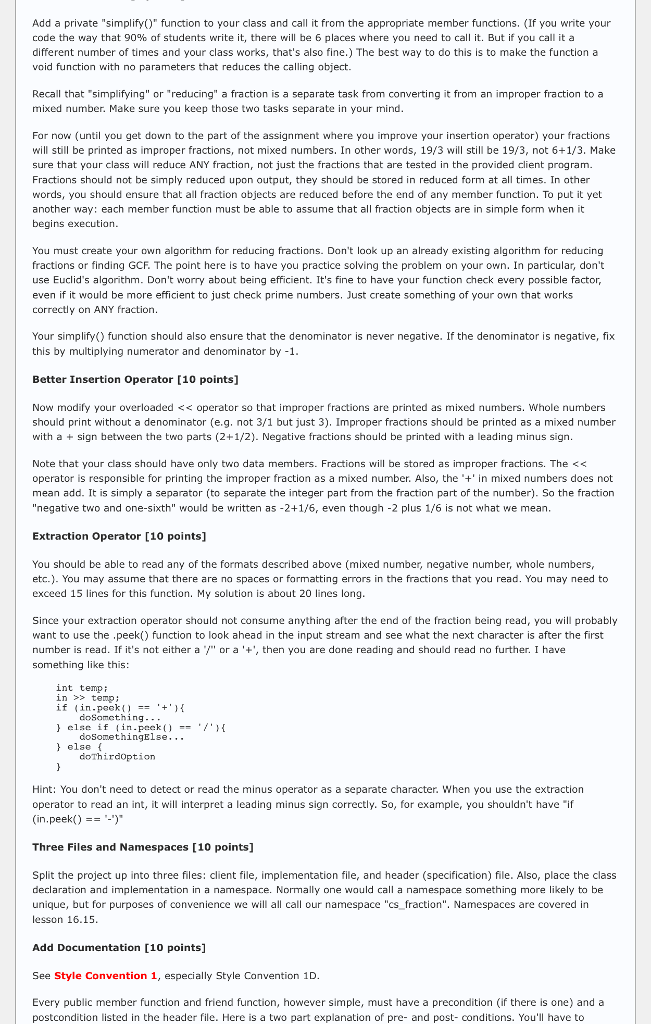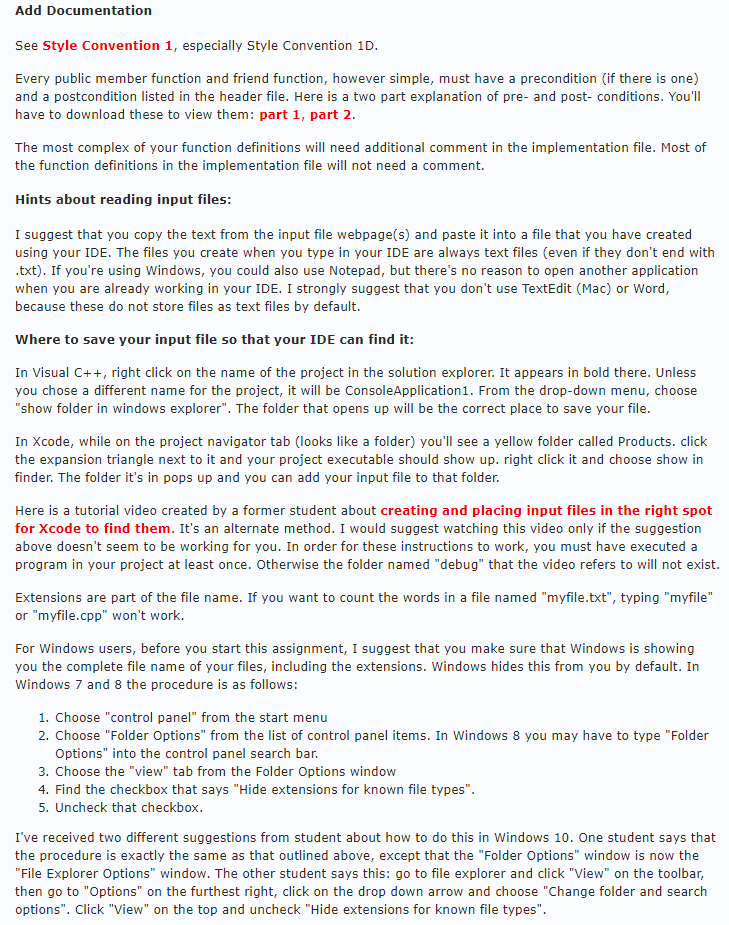Client Program:

```#include
#include "Fraction.h"
#include
#include
#include
using namespace std;
using namespace cs_Fraction;

void BasicTest();
void RelationTest();
void BinaryMathTest();
void MathAssignTest();
bool eof(ifstream& in);
string boolString(bool convertMe);

int main()
{
BasicTest();
RelationTest();
BinaryMathTest();
MathAssignTest();
}

void BasicTest()
{
cout << "n----- Testing basic Fraction creation & printingn";
cout << "(Fractions should be in reduced form, and as mixed numbers.)n";

const Fraction fr[] = {Fraction(4, 8), Fraction(-15,21),
Fraction(10), Fraction(12, -3),
Fraction(), Fraction(28, 6), Fraction(0, 12)};

for (int i = 0; i < 7; i++){
cout << "Fraction [" << i <<"] = " << fr[i] << endl;
}

cout << "n----- Now reading Fractions from filen";
ifstream in("Fraction.txt");
assert(in);
while (!eof(in)) {
Fraction f;
if (in.peek() == '#') {
in.ignore(128, 'n');                       //skip this line, it's a comment
} else {
in >> f;
cout << "Read Fraction = " << f << endl;
}
}
}

bool eof(ifstream& in)
{
char ch;
in >> ch;
in.putback(ch);
return !in;
}

string boolString(bool convertMe) {
if (convertMe) {
return "true";
} else {
return "false";
}
}

void RelationTest()
{
cout << "n----- Testing relational operators between Fractionsn";

const Fraction fr[] =  {Fraction(3, 6), Fraction(1,2), Fraction(-15,30),
Fraction(1,10), Fraction(0,1), Fraction(0,2)};

for (int i = 0; i < 5; i++) {
cout << "Comparing " << fr[i] << " to " << fr[i+1] << endl;
cout << "tIs left < right? " << boolString(fr[i] < fr[i+1]) << endl;
cout << "tIs left <= right? " << boolString(fr[i] <= fr[i+1]) << endl;
cout << "tIs left > right? " << boolString(fr[i] > fr[i+1]) << endl;
cout << "tIs left >= right? " << boolString(fr[i] >= fr[i+1]) << endl;
cout << "tDoes left == right? " << boolString(fr[i] == fr[i+1]) << endl;
cout << "tDoes left != right ? " << boolString(fr[i] != fr[i+1]) << endl;
}

cout << "n----- Testing relations between Fractions and integersn";
Fraction f(-3,6);
int num = 2;
cout << "Comparing " << f << " to " << num << endl;
cout << "tIs left < right? " << boolString(f < num) << endl;
cout << "tIs left <= right? " << boolString(f <= num) << endl;
cout << "tIs left > right? " << boolString(f > num) << endl;
cout << "tIs left >= right? " << boolString(f >= num) << endl;
cout << "tDoes left == right? " << boolString(f == num) << endl;
cout << "tDoes left != right ? " << boolString(f != num) << endl;

Fraction g(1,4);
num = -3;
cout << "Comparing " << num << " to " << g << endl;
cout << "tIs left < right? " << boolString(num < g) << endl;
cout << "tIs left <= right? " << boolString(num <= g) << endl;
cout << "tIs left > right? " << boolString(num > g) << endl;
cout << "tIs left >= right? " << boolString(num >= g) << endl;
cout << "tDoes left == right? " << boolString(num == g) << endl;
cout << "tDoes left != right ? " << boolString(num != g) << endl;
}

void BinaryMathTest()
{
cout << "n----- Testing binary arithmetic between Fractionsn";

const Fraction fr[] = {Fraction(1, 6), Fraction(1,3),
Fraction(-2,3), Fraction(5), Fraction(-4,3)};

for (int i = 0; i < 4; i++) {
cout << fr[i] << " + " << fr[i+1] << " = " << fr[i] + fr[i+1] << endl;
cout << fr[i] << " - " << fr[i+1] << " = " << fr[i] - fr[i+1] << endl;
cout << fr[i] << " * " << fr[i+1] << " = " << fr[i] * fr[i+1] << endl;
cout << fr[i] << " / " << fr[i+1] << " = " << fr[i] / fr[i+1] << endl;
}

cout << "n----- Testing arithmetic between Fractions and integersn";
Fraction f(-1, 2);
int num = 4;
cout << f << " + " << num << " = " << f + num << endl;
cout << f << " - " << num << " = " << f - num << endl;
cout << f << " * " << num << " = " << f * num << endl;
cout << f << " / " << num << " = " << f / num << endl;

Fraction g(-1, 2);
num = 3;
cout << num << " + " << g << " = " << num + g << endl;
cout << num << " - " << g << " = " << num - g << endl;
cout << num << " * " << g << " = " << num * g << endl;
cout << num << " / " << g << " = " << num / g << endl;
}

void MathAssignTest()
{
cout << "n----- Testing shorthand arithmetic assignment on Fractionsn";

Fraction fr[] = {Fraction(1, 6), Fraction(4),
Fraction(-1,2), Fraction(5)};

for (int i = 0; i < 3; i++) {
cout << fr[i] << " += " << fr[i+1] << " = ";
cout << (fr[i] += fr[i+1]) << endl;
cout << fr[i] << " -= " << fr[i+1] << " = ";
cout << (fr[i] -= fr[i+1]) << endl;
cout << fr[i] << " *= " << fr[i+1] << " = ";
cout << (fr[i] *= fr[i+1]) << endl;
cout << fr[i] << " /= " << fr[i+1] << " = ";
cout << (fr[i] /= fr[i+1]) << endl;
}

cout << "n----- Testing shorthand arithmetic assignment using integersn";
Fraction f(-1, 3);
int num = 3;
cout << f << " += " << num << " = ";
cout << (f += num) << endl;
cout << f << " -= " << num << " = ";
cout << (f -= num) << endl;
cout << f << " *= " << num << " = ";
cout << (f *= num) << endl;
cout << f << " /= " << num << " = ";
cout << (f /= num) << endl;

cout << "n----- Testing increment/decrement prefix and postfixn";
Fraction g(-1, 3);
cout << "Now g = " << g << endl;
cout << "g++ = " << g++ << endl;
cout << "Now g = " << g << endl;
cout << "++g = " << ++g << endl;
cout << "Now g = " << g << endl;
cout << "g-- = " << g-- << endl;
cout << "Now g = " << g << endl;
cout << "--g = " << --g << endl;
cout << "Now g = " << g << endl;
}
```

Frac data:

```# This file shows the patterns your Fraction class needs to be able to
# read.  A Fraction may be just a single integer, two integers separated by
# a slash, or a mixed number which consists of an integer, followed by a +
# and then two integers with a slash.  A minus sign may appear in the
# very first character to indicate the whole Fraction is negative.
# No white space is allowed in between the component parts of a Fraction.
#
1/3
3/6
3072/4096
-4/5
12/2
5
-8
21/15
-50/3
1+1/4
1+5/5
-4+3/12
-10+10/12
```

Output:

```----- Testing basic Fraction creation & printing
(Fractions should be in reduced form, and as mixed numbers.)
Fraction  = 1/2
Fraction  = -5/7
Fraction  = 10
Fraction  = -4
Fraction  = 0
Fraction  = 4+2/3
Fraction  = 0

----- Now reading Fractions from file

----- Testing relational operators between Fractions
Comparing 1/2 to 1/2
Is left < right? false
Is left <= right? true
Is left > right? false
Is left >= right? true
Does left == right? true
Does left != right ? false
Comparing 1/2 to -1/2
Is left < right? false
Is left <= right? false
Is left > right? true
Is left >= right? true
Does left == right? false
Does left != right ? true
Comparing -1/2 to 1/10
Is left < right? true
Is left <= right? true
Is left > right? false
Is left >= right? false
Does left == right? false
Does left != right ? true
Comparing 1/10 to 0
Is left < right? false
Is left <= right? false
Is left > right? true
Is left >= right? true
Does left == right? false
Does left != right ? true
Comparing 0 to 0
Is left < right? false
Is left <= right? true
Is left > right? false
Is left >= right? true
Does left == right? true
Does left != right ? false

----- Testing relations between Fractions and integers
Comparing -1/2 to 2
Is left < right? true
Is left <= right? true
Is left > right? false
Is left >= right? false
Does left == right? false
Does left != right ? true
Comparing -3 to 1/4
Is left < right? true
Is left <= right? true
Is left > right? false
Is left >= right? false
Does left == right? false
Does left != right ? true

----- Testing binary arithmetic between Fractions
1/6 + 1/3 = 1/2
1/6 - 1/3 = -1/6
1/6 * 1/3 = 1/18
1/6 / 1/3 = 1/2
1/3 + -2/3 = -1/3
1/3 - -2/3 = 1
1/3 * -2/3 = -2/9
1/3 / -2/3 = -1/2
-2/3 + 5 = 4+1/3
-2/3 - 5 = -5+2/3
-2/3 * 5 = -3+1/3
-2/3 / 5 = -2/15
5 + -1+1/3 = 3+2/3
5 - -1+1/3 = 6+1/3
5 * -1+1/3 = -6+2/3
5 / -1+1/3 = -3+3/4

----- Testing arithmetic between Fractions and integers
-1/2 + 4 = 3+1/2
-1/2 - 4 = -4+1/2
-1/2 * 4 = -2
-1/2 / 4 = -1/8
3 + -1/2 = 2+1/2
3 - -1/2 = 3+1/2
3 * -1/2 = -1+1/2
3 / -1/2 = -6

----- Testing shorthand arithmetic assignment on Fractions
1/6 += 4 = 4+1/6
4+1/6 -= 4 = 1/6
1/6 *= 4 = 2/3
2/3 /= 4 = 1/6
4 += -1/2 = 3+1/2
3+1/2 -= -1/2 = 4
4 *= -1/2 = -2
-2 /= -1/2 = 4
-1/2 += 5 = 4+1/2
4+1/2 -= 5 = -1/2
-1/2 *= 5 = -2+1/2
-2+1/2 /= 5 = -1/2

----- Testing shorthand arithmetic assignment using integers
-1/3 += 3 = 2+2/3
2+2/3 -= 3 = -1/3
-1/3 *= 3 = -1
-1 /= 3 = -1/3

----- Testing increment/decrement prefix and postfix
Now g = -1/3
g++ = -1/3
Now g = 2/3
++g = 1+2/3
Now g = 1+2/3
g-- = 1+2/3
Now g = 2/3
--g = -1/3
Now g = -1/3
```

main.cpp
————————————————-
#include <iostream>
#include <string>
using namespace std;

class fraction {

//variables
private: int numerator;
int denominator;

//functions
public:
// default constructor
fraction();
//constructor with one agrument
fraction(int);
//constructor with two agruments
fraction(int, int);

friend std::ostream& operator<<(std::ostream &out, const fraction &inFraction);

//Compare operators
friend bool operator== (const fraction& inLeft, const fraction& inRight);
friend bool operator!= (const fraction& inLeft, const fraction& inRight);
friend bool operator> (const fraction& inLeft, const fraction& inRight);
friend bool operator>= (const fraction& inLeft, const fraction& inRight);
friend bool operator< (const fraction& inLeft, const fraction& inRight);
friend bool operator<= (const fraction& inLeft, const fraction& inRight);

//Operations operators
friend fraction operator+ (const fraction& inLeft, const fraction& inRight);
friend fraction operator- (const fraction& inLeft, const fraction& inRight);
friend fraction operator* (const fraction& inLeft, const fraction& inRight);
friend fraction operator/ (const fraction& inLeft, const fraction& inRight);

//
fraction operator+= (const fraction& inFraction);
fraction operator-= (const fraction& inFraction);
fraction operator*= (const fraction& inFraction);
fraction operator/= (const fraction& inFraction);

//Prefix and Postfix
fraction operator++ ();
fraction operator++ (int);
fraction operator– ();
fraction operator– (int);

};

//**************************************CONSTRUCTORS***************************************************
/*
* default constructor
*/
fraction:: fraction() {
numerator = 0;
denominator = 1;
}

/*
* constructor with one agrument
*/
fraction:: fraction(int number) {
numerator = number;
denominator = 1;
}

/*
* constructor with two agruments
*/
fraction:: fraction(int inNumerator,int inDenominator)
{
numerator = inNumerator;

if(inDenominator == 0)
{
denominator = 1;
}
else
{
denominator = inDenominator;
}
}

//**********************************OUT OPERATOR***************************************
ostream& operator<< (ostream& out, const fraction& inFraction) {
return out << ‘(‘ << inFraction.numerator << ‘/’ << inFraction.denominator << ‘)’;
}

//*************************************COMPARE OPERATORS*********************************************
/*
*
*/
bool operator< (const fraction& inLeft, const fraction& inRight)
{
return (inLeft.numerator * inRight.denominator < inRight.numerator * inLeft.denominator);
}

/*
*
*/
bool operator<= (const fraction& inLeft, const fraction& inRight)
{
return (inLeft.numerator * inRight.denominator <= inRight.numerator * inLeft.denominator);
}

/*
*
*/
bool operator> (const fraction& inLeft, const fraction& inRight)
{
return (inLeft.numerator * inRight.denominator > inRight.numerator * inLeft.denominator);
}

/*
*
*/
bool operator>= (const fraction& inLeft, const fraction& inRight)
{
return (inLeft.numerator * inRight.denominator >= inRight.numerator * inLeft.denominator);
}

/*
*
*/
bool operator== (const fraction& inLeft, const fraction& inRight)
{
return (inLeft.numerator * inRight.denominator == inRight.numerator * inLeft.denominator);
}

/*
*
*/
bool operator!= (const fraction& inLeft, const fraction& inRight)
{
return (inLeft.numerator * inRight.denominator != inRight.numerator * inLeft.denominator);
}

//***************************************Operators*****************************************
/*
*
*/
fraction operator+ (const fraction& inLeft, const fraction& inRight) {
fraction result(inLeft.numerator * inRight.denominator + inRight.numerator * inLeft.denominator,
inLeft.denominator * inRight.denominator );
return result;
}

/*
*
*/
fraction operator- (const fraction& inLeft, const fraction& inRight) {
fraction result(inLeft.numerator * inRight.denominator –
inRight.numerator * inLeft.denominator,
inLeft.denominator * inRight.denominator);
return result;
}

/*
*
*/
fraction operator* (const fraction& inLeft, const fraction& inRight) {
fraction result(inLeft.numerator * inRight.numerator,
inLeft.denominator * inRight.denominator);
return result;
}

/*
*
*/
fraction operator/ (const fraction& inLeft, const fraction& inRight) {
fraction result(inLeft.numerator * inRight.denominator,
inLeft.denominator * inRight.numerator);
return result;
}

/*
*
*/
fraction fraction::operator+=(const fraction &inFraction) {
*this = *this + inFraction;
return *this;
}

/*
*
*/
fraction fraction::operator-=(const fraction &inFraction) {
*this = *this – inFraction;
return *this;
}

/*
*
*/
fraction fraction::operator*=(const fraction &inFraction) {
*this = *this * inFraction;
return *this;
}

/*
*
*/
fraction fraction::operator/=(const fraction &inFraction) {
*this = *this / inFraction;
return *this;
}

//*****************************PREFIX AND POSTFIX**************************************
/*
*
*/
fraction fraction::operator++ () {
numerator += denominator;
return *this;
}

/*
*
*/
fraction fraction::operator++ (int) {
fraction temp(numerator, denominator);
numerator += denominator;
return temp;
}

/*
*
*/
fraction fraction::operator– () {
numerator -= denominator;
return *this;
}

/*
*
*/
fraction fraction::operator– (int) {
fraction temp(numerator, denominator);
numerator -= denominator;
return temp;
}

//*****************************************TEST***********************************

void BasicTest();
void RelationTest();
void BinaryMathTest();
void MathAssignTest();
string boolString(bool convertMe);

int main()
{
BasicTest();
RelationTest();
BinaryMathTest();
MathAssignTest();
}

void BasicTest()
{
cout << “n—– Testing basic fraction creation & printingn”;

const fraction fr[] = {fraction(4, 8), fraction(-15,21),
fraction(10), fraction(12, -3),
fraction(), fraction(28, 6), fraction(0, 12)};

for (int i = 0; i < 7; i++){
cout << “fraction [” << i <<“] = ” << fr[i] << endl;
}

}

string boolString(bool convertMe) {
if (convertMe) {
return “true”;
} else {
return “false”;
}
}

void RelationTest()
{
cout << “n—– Testing relational operators between fractionsn”;

const fraction fr[] = {fraction(3, 6), fraction(1,2), fraction(-15,30),
fraction(1,10), fraction(0,1), fraction(0,2)};

for (int i = 0; i < 5; i++) {
cout << “Comparing ” << fr[i] << ” to ” << fr[i+1] << endl;
cout << “tIs left < right? ” << boolString(fr[i] < fr[i+1]) << endl;
cout << “tIs left <= right? ” << boolString(fr[i] <= fr[i+1]) << endl;
cout << “tIs left > right? ” << boolString(fr[i] > fr[i+1]) << endl;
cout << “tIs left >= right? ” << boolString(fr[i] >= fr[i+1]) << endl;
cout << “tDoes left == right? ” << boolString(fr[i] == fr[i+1]) << endl;
cout << “tDoes left != right ? ” << boolString(fr[i] != fr[i+1]) << endl;
}

cout << “n—– Testing relations between fractions and integersn”;
fraction f(-3,6);
int num = 2;
cout << “Comparing ” << f << ” to ” << num << endl;
cout << “tIs left < right? ” << boolString(f < num) << endl;
cout << “tIs left <= right? ” << boolString(f <= num) << endl;
cout << “tIs left > right? ” << boolString(f > num) << endl;
cout << “tIs left >= right? ” << boolString(f >= num) << endl;
cout << “tDoes left == right? ” << boolString(f == num) << endl;
cout << “tDoes left != right ? ” << boolString(f != num) << endl;

fraction g(1,4);
num = -3;
cout << “Comparing ” << num << ” to ” << g << endl;
cout << “tIs left < right? ” << boolString(num < g) << endl;
cout << “tIs left <= right? ” << boolString(num <= g) << endl;
cout << “tIs left > right? ” << boolString(num > g) << endl;
cout << “tIs left >= right? ” << boolString(num >= g) << endl;
cout << “tDoes left == right? ” << boolString(num == g) << endl;
cout << “tDoes left != right ? ” << boolString(num != g) << endl;
}

void BinaryMathTest()
{
cout << “n—– Testing binary arithmetic between fractionsn”;

const fraction fr[] = {fraction(1, 6), fraction(1,3),
fraction(-2,3), fraction(5), fraction(-4,3)};

for (int i = 0; i < 4; i++) {
cout << fr[i] << ” + ” << fr[i+1] << ” = ” << fr[i] + fr[i+1] << endl;
cout << fr[i] << ” – ” << fr[i+1] << ” = ” << fr[i] – fr[i+1] << endl;
cout << fr[i] << ” * ” << fr[i+1] << ” = ” << fr[i] * fr[i+1] << endl;
cout << fr[i] << ” / ” << fr[i+1] << ” = ” << fr[i] / fr[i+1] << endl;
}

cout << “n—– Testing arithmetic between fractions and integersn”;
fraction f(-1, 2);
int num = 4;
cout << f << ” + ” << num << ” = ” << f + num << endl;
cout << f << ” – ” << num << ” = ” << f – num << endl;
cout << f << ” * ” << num << ” = ” << f * num << endl;
cout << f << ” / ” << num << ” = ” << f / num << endl;

fraction g(-1, 2);
num = 3;
cout << num << ” + ” << g << ” = ” << num + g << endl;
cout << num << ” – ” << g << ” = ” << num – g << endl;
cout << num << ” * ” << g << ” = ” << num * g << endl;
cout << num << ” / ” << g << ” = ” << num / g << endl;
}

void MathAssignTest()
{
cout << “n—– Testing shorthand arithmetic assignment on fractionsn”;

fraction fr[] = {fraction(1, 6), fraction(4),
fraction(-1,2), fraction(5)};

for (int i = 0; i < 3; i++) {
cout << fr[i] << ” += ” << fr[i+1] << ” = “;
cout << (fr[i] += fr[i+1]) << endl;
cout << fr[i] << ” -= ” << fr[i+1] << ” = “;
cout << (fr[i] -= fr[i+1]) << endl;
cout << fr[i] << ” *= ” << fr[i+1] << ” = “;
cout << (fr[i] *= fr[i+1]) << endl;
cout << fr[i] << ” /= ” << fr[i+1] << ” = “;
cout << (fr[i] /= fr[i+1]) << endl;
}

cout << “n—– Testing shorthand arithmetic assignment using integersn”;
fraction f(-1, 3);
int num = 3;
cout << f << ” += ” << num << ” = “;
cout << (f += num) << endl;
cout << f << ” -= ” << num << ” = “;
cout << (f -= num) << endl;
cout << f << ” *= ” << num << ” = “;
cout << (f *= num) << endl;
cout << f << ” /= ” << num << ” = “;
cout << (f /= num) << endl;

cout << “n—– Testing increment/decrement prefix and postfixn”;
fraction g(-1, 3);
cout << “Now g = ” << g << endl;
cout << “g++ = ” << g++ << endl;
cout << “Now g = ” << g << endl;
cout << “++g = ” << ++g << endl;
cout << “Now g = ” << g << endl;
cout << “g– = ” << g– << endl;
cout << “Now g = ” << g << endl;
cout << “–g = ” << –g << endl;
cout << “Now g = ” << g << endl;
}

———————————————————————–
fraction.cpp
—————————————-
#include “fraction.h”

namespace cs_fraction {

using namespace std;

fraction::fraction(int inNumerator, int inDenominator) {
assert(inDenominator != 0);
numerator = inNumerator;
denominator = inDenominator;
this->simplify();
}

ostream& operator<< (ostream& out, const fraction& inFraction) {

if (abs(inFraction.numerator) >= abs(inFraction.denominator)) {
out << inFraction.numerator / inFraction.denominator;

if (inFraction.numerator % inFraction.denominator != 0) {
out << “+” << abs(inFraction.numerator % inFraction.denominator) << “/” <<
inFraction.denominator;
}
}

else if (inFraction.numerator == 0) {
out << 0;
}

else {
out << inFraction.numerator << “/” << inFraction.denominator;
}

return out;
}

//Takes user input of mixed numbers, negative numbers, whole numbers or fractions
//and stores them in fraction f.
istream& operator>> (istream& in, fraction& inFraction)
{
int temp;
in >> temp;
if (in.peek() == ‘+’) {

in.ignore();
in >> inFraction.numerator;
in.ignore();
in >> inFraction.denominator;

if(temp < 0) {

temp *= -1;
inFraction.numerator += temp * inFraction.denominator;
inFraction.numerator *= -1;
}

else {

inFraction.numerator += temp * inFraction.denominator;
}
}
else if (in.peek() == ‘/’) {

in.ignore();
in >> inFraction.denominator;
inFraction.numerator = temp;
}
else {

inFraction.numerator = temp;
inFraction.denominator = 1;
}

inFraction.simplify();
return in;
}

bool operator< (const fraction& inLeft, const fraction& inRight) {

return (inLeft.numerator * inRight.denominator < inRight.numerator * inLeft.denominator);
}

bool operator<= (const fraction& inLeft, const fraction& inRight) {

return (inLeft.numerator * inRight.denominator <= inRight.numerator * inLeft.denominator);
}

bool operator> (const fraction& inLeft, const fraction& inRight) {

return (inLeft.numerator * inRight.denominator > inRight.numerator * inLeft.denominator);
}

bool operator>= (const fraction& inLeft, const fraction& inRight) {

return (inLeft.numerator * inRight.denominator >= inRight.numerator * inLeft.denominator);
}

bool operator== (const fraction& inLeft, const fraction& inRight) {

return (inLeft.numerator * inRight.denominator == inRight.numerator * inLeft.denominator);
}

bool operator!= (const fraction& inLeft, const fraction& inRight) {

return (inLeft.numerator * inRight.denominator != inRight.numerator * inLeft.denominator);
}

fraction operator+ (const fraction& inLeft, const fraction& inRight) {

fraction result(inLeft.numerator * inRight.denominator +
inRight.numerator * inLeft.denominator,
inLeft.denominator * inRight.denominator );
return result;
}

fraction operator- (const fraction& inLeft, const fraction& inRight) {

fraction result(inLeft.numerator * inRight.denominator –
inRight.numerator * inLeft.denominator,
inLeft.denominator * inRight.denominator);
return result;
}

fraction operator* (const fraction& inLeft, const fraction& inRight) {

fraction result(inLeft.numerator * inRight.numerator,
inLeft.denominator * inRight.denominator);
return result;
}

fraction operator/ (const fraction& inLeft, const fraction& inRight) {

fraction result(inLeft.numerator * inRight.denominator,
inLeft.denominator * inRight.numerator);
return result;
}

fraction fraction::operator+=(const fraction &inFraction) {

*this = *this + inFraction;
return *this;
}

fraction fraction::operator-=(const fraction &inFraction) {

*this = *this – inFraction;
return *this;
}

fraction fraction::operator*=(const fraction &inFraction) {

*this = *this * inFraction;
return *this;
}

fraction fraction::operator/=(const fraction &inFraction) {

*this = *this / inFraction;
return *this;
}

fraction fraction::operator++ () {

numerator += denominator;
return *this;
}

fraction fraction::operator++ (int) {

fraction temp(numerator, denominator);
numerator += denominator;
return temp;
}

fraction fraction::operator– () {

numerator -= denominator;
return *this;
}

fraction fraction::operator– (int) {

fraction temp(numerator, denominator);
numerator -= denominator;
return temp;
}

void fraction::simplify() {

for (int i = denominator; i > 1; i–) {

if ((numerator % i == 0) && (denominator % i == 0)) {

numerator = numerator / i;
denominator = denominator / i;
}
}
if(denominator < 0) {

denominator *= -1;
numerator *= -1;
}
}
}
————————————————————————-
fraction.h
——————————————-
#include <iostream>
#include <cassert>
#include <cmath>

namespace cs_fraction {

class fraction {

//variables
private: int numerator;
int denominator;
void simplify();

//functions
public:
fraction(int inNumerator = 0, int inDenominator = 1);

friend std::ostream& operator<<(std::ostream& out, const fraction& inFraction);
friend std::istream& operator>>(std::istream& in, fraction& inFraction);

friend bool operator== (const fraction& inLeft, const fraction& inRight);
friend bool operator!= (const fraction& inLeft, const fraction& inRight);
friend bool operator> (const fraction& inLeft, const fraction& inRight);
friend bool operator>= (const fraction& inLeft, const fraction& inRight);
friend bool operator< (const fraction& inLeft, const fraction& inRight);
friend bool operator<= (const fraction& inLeft, const fraction& inRight);

friend fraction operator+ (const fraction& inLeft, const fraction& inRight);
friend fraction operator- (const fraction& inLeft, const fraction& inRight);
friend fraction operator* (const fraction& inLeft, const fraction& inRight);
friend fraction operator/ (const fraction& inLeft, const fraction& inRight);

fraction operator+= (const fraction& inFraction);
fraction operator-= (const fraction& inFraction);
fraction operator*= (const fraction& inFraction);
fraction operator/= (const fraction& inFraction);

fraction operator++ ();
fraction operator++ (int);
fraction operator– ();
fraction operator– (int);

};   //end class
} //end namespace
—————————————————————————————–
fraction.txt
———————————-
1/3
3/6
3072/4096
-4/5
12/2
5
-8
21/15
-50/3
1+1/4
1+5/5
-4+3/12
-10+10/12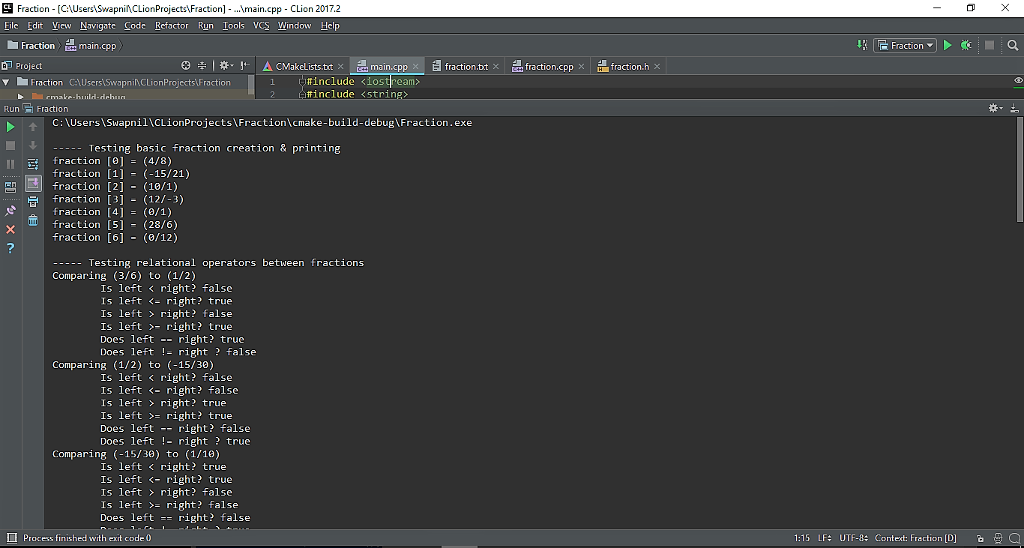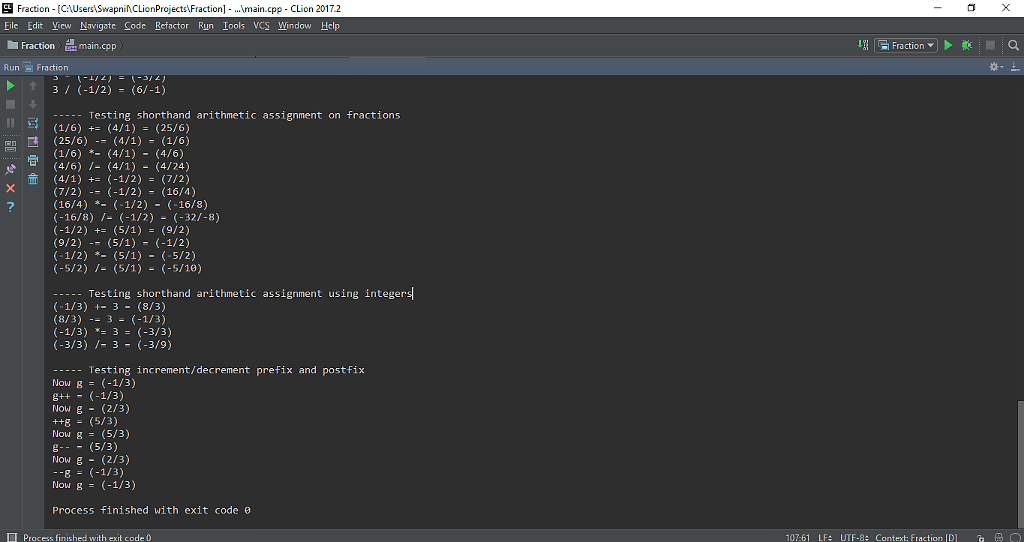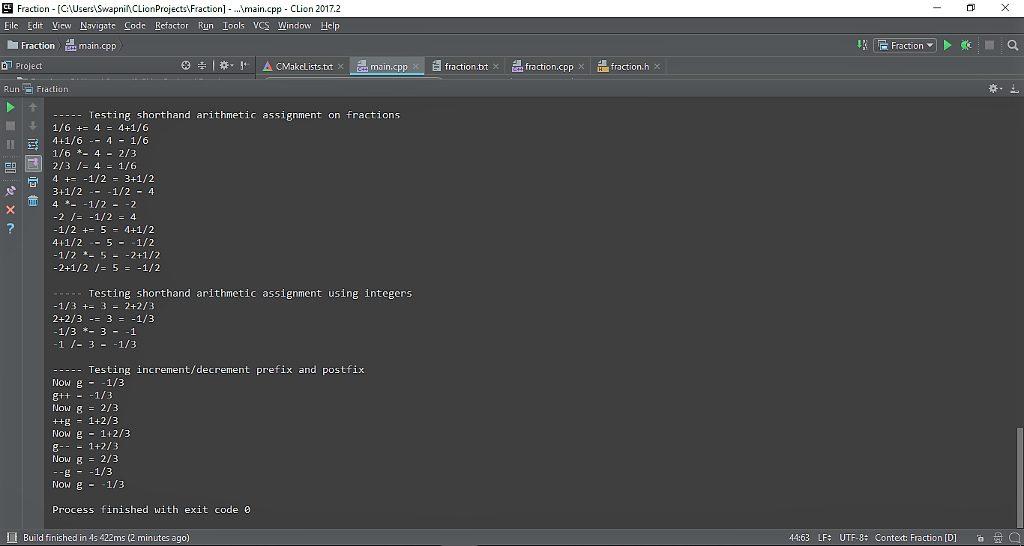Pages (550 words)
Approximate price: -

Help Me Write My Essay - Reasons:Best Online Essay Writing Service

We strive to give our customers the best online essay writing experience. We Make sure essays are submitted on time and all the instructions are followed.Our Writers are Experienced and Professional

Our essay writing service is founded on professional writers who are on stand by to help you any time.Free Revision Fo all Essays

Sometimes you may require our writers to add on a point to make your essay as customised as possible, we will give you unlimited times to do this. And we will do it for free.Timely Essay(s)

We understand the frustrations that comes with late essays and our writers are extra careful to not violate this term. Our support team is always engauging our writers to help you have your essay ahead of time.Customised Essays &100% Confidential

Our Online writing Service has zero torelance for plagiarised papers. We have plagiarism checking tool that generate plagiarism reports just to make sure you are satisfied.Try it now!

## Calculate the price of your order

Total price:
\$0.00

How it works?Fill in the order form and provide all details of your assignment.Proceed with the payment

Choose the payment system that suits you most.HOW OUR ONLINE ESSAY WRITING SERVICE WORKS

Let us write that nagging essay.By clicking on the "PLACE ORDER" button, tell us your requires. Be precise for an accurate customised essay. You may also upload any reading materials where applicable.Pick A & Writer

Our ordering form will provide you with a list of writers and their feedbacks. At step 2, its time select a writer. Our online agents are on stand by to help you just in case.Editing (OUR PART)

At this stage, our editor will go through your essay and make sure your writer did meet all the instructions.